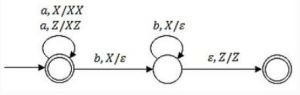## Push-Down-Automata

 Question 1

Consider the transition diagram of a PDA given below with input alphabet Σ = {a,b} and stack alphabet Γ = {X,Z}. Z is the initial stack symbol. Let L denote the language accepted by the PDA.Which one of the following is TRUE?

 A L = {an bn│n ≥ 0} and is not accepted by any ﬁnite automata B L = {an |n≥0} ∪ {anbn|n ≥ 0} and is not accepted by any deterministic PDA C L is not accepted by any Turing machine that halts on every input D L = {an |n ≥ 0} ∪ {an bn |n ≥ 0} and is deterministic context-free
Theory-of-Computation       Push-Down-Automata       GATE 2016 [Set-1]       Video-Explanation
Question 1 Explanation:
In this PDA, we can give labels to state as q0, q1, q2
where q0 and q2 are final states.
This PDA accepts the string by both ways i.e. by using q0 accepts as final state and by using q2 it accepts as empty stack.
Since q0 is initial as well as final state, so it can accept any number of a’s (including zero a’s) and by using q2 as empty stack it accept strings which has equal number of a’s and b’s (b’s comes after a’s).
Hence, L = {an | n≥0} ∪ { an bn | n≥0}.
 Question 2

Give a deterministic PDA for the language L = {ancb2n|n ≥ 1} over the alphabet Σ = {a,b,c}. Specify the acceptance state.

 A Theory Explanation is given below.
Theory-of-Computation       Push-Down-Automata       GATE 2001
 Question 3

Let LD be the set of all languages accepted by a PDA by final state and LE the set of all languages accepted by empty stack. Which of the following is true?

 A LD = LE B LD ⊃ LE C LE = LD D None of the above
Theory-of-Computation       Push-Down-Automata       GATE 1999
Question 3 Explanation:
For any PDA which can be accepted by final state, there is an equivalent PDA which can also be accepted by an empty stack and for any PDA which can be accepted by an empty stack, there is an equivalent PDA which can be accepted by final state.
There are 3 questions to complete.

Register Now